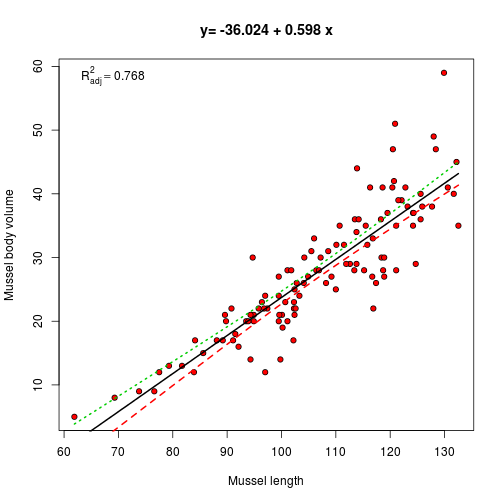# Correlation and regression analysis

Place this file in the folder with your data. Write the names of your own data file below instead of the one shown.Then compile the script using R studio. It will build a data report that will guide you through the analysis.

``````d <- read.csv("filename.csv")  ## Put your own file name here
xtext <- "Mussel length"
ytext <- "Mussel body volume"
source("StatsExpert.R")
``````

StatsExpert is a script written in R that has been designed to help you to choose the right analysis for your own data by writing out suggestions and running some of the most appropriate analyses.

It is not guaranteed to be foolproof. In most cases the suggestions made will be reasonable, but if the data are very unusual some of the statements might appear constradictory. Statistical analysis is not an exact science that can be turned into simple rules. However the script has been designed to help you avoid the worst pitfalls.

The StatExpert script will first run through the flow diagram shown below.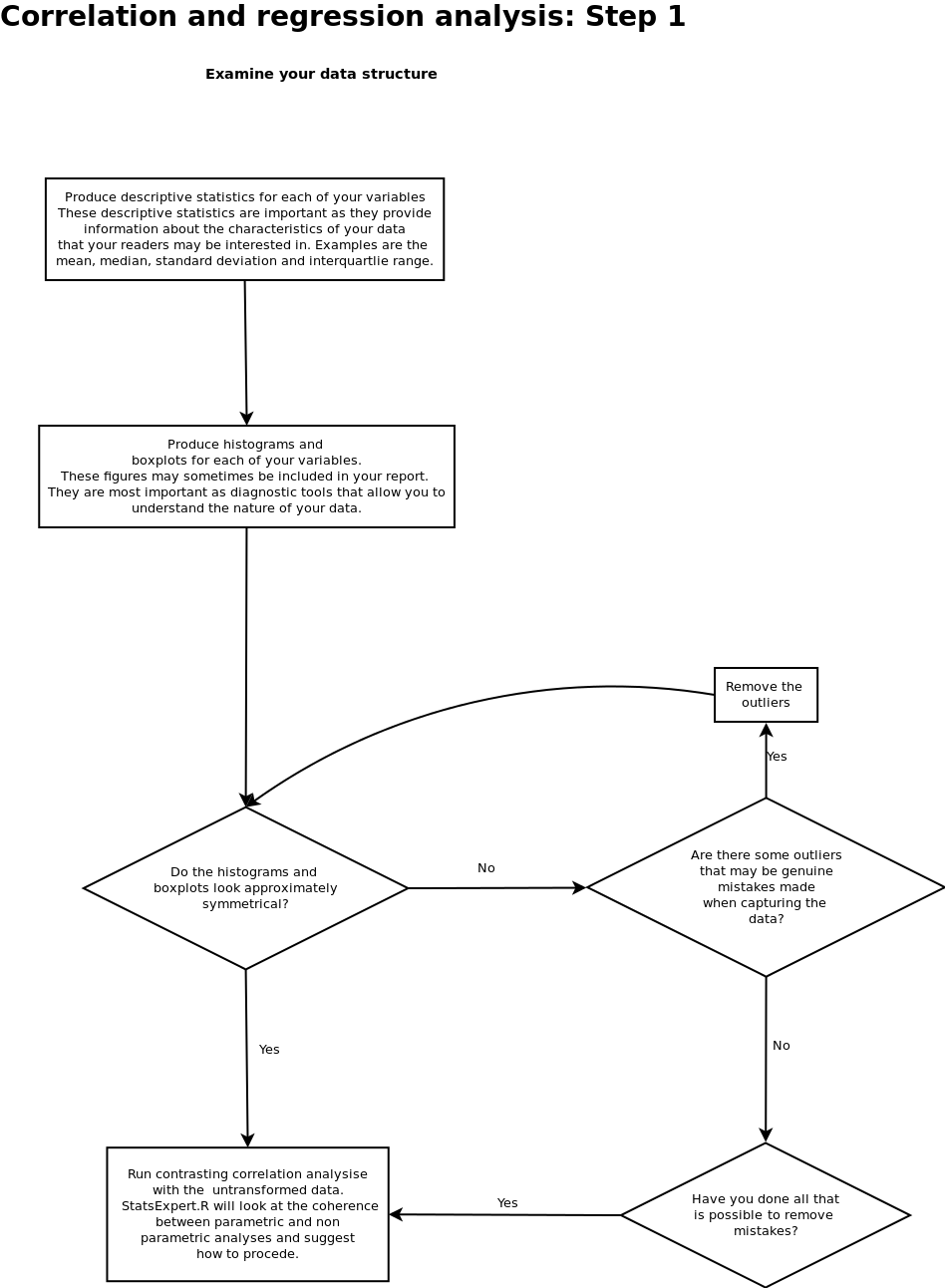## Descriptives

You will need to provide descriptive statistics for each variable regardless of the outcome of the rest of the analysis.

``````summary(d)
``````
``````##      Lshell         BTVolume
##  Min.   : 61.9   Min.   : 5.0
##  1st Qu.: 97.0   1st Qu.:21.0
##  Median :106.9   Median :28.0
##  Mean   :106.8   Mean   :27.8
##  3rd Qu.:118.7   3rd Qu.:35.0
##  Max.   :132.6   Max.   :59.0
``````

This table shows you the minimum, maximum and interquartile range. You should also look at the mean and median for each variable. If there is a big difference between them it could be an indication of skew.

## Looking at the distribution of each variable

It is very important to produce boxplots and histograms for each variable. You should look very carefully at these figures in order to spot potentially problems with the analysis.

For correlation and regression analysis the raw variables do not have to be normally distributed. However they should usually be more or less symmetrically distributed. So we need to check for skew, and we also need to look carefully at the most extreme values (outliers)

The StatsExpert script will try to help to find problems, but always be aware that it cannot be expected to solve all the issues nor can it know why some of the values may be outside the expected range.

``````
par(mfrow = c(2, 2))
hist(x, col = "grey", main = xtext)
boxplot(x, col = "grey", main = xtext)
hist(y, col = "grey", main = ytext)
boxplot(y, col = "grey", main = ytext)
``````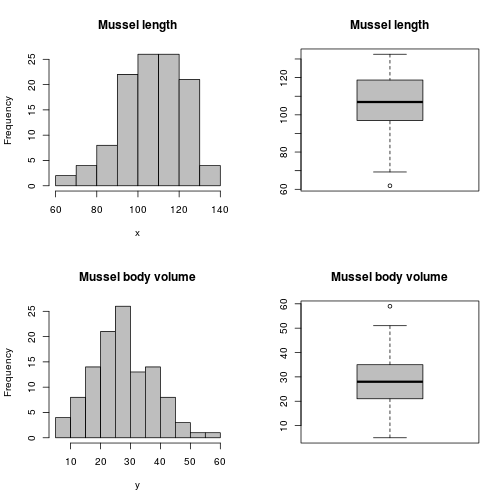``````
``````

Things to watch for are outliers and skew. If a boxplot has many points beyond the whiskers then you may have problems with outliers. If the histogram does not look symmetrical then you have a potential problem with skew. These two issues go together and are very difficult to separate cleanly, so only use StatsExpert as a rough guide. Skewed data has many outliers, and outliers lead to skew. However watch out foe outliers that are really just mistakes. These are best removed from your data.

To help you to interpret these figures and get an idea of what you should be looking for examine the examples below. Hopefully your figures are closer to the blue histograms and boxplots than the red ones.If the data are seriously skewed you have not necessarily made a mistake! Data like these are very commonly found in ecology. StatsExpert will try to help you interpret the data that you have collected.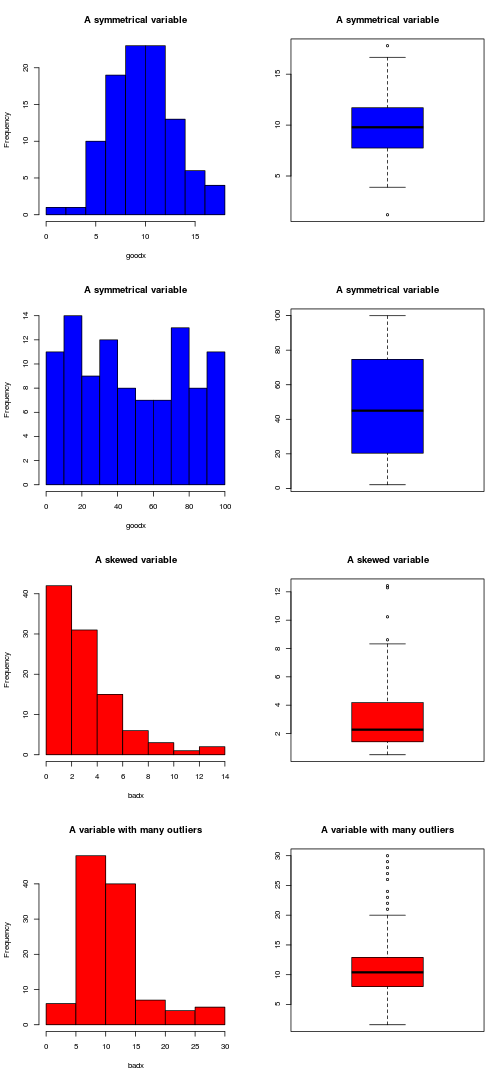## Automated checks for skew and outliers

StatsExpert will have a look at your data for you and do its best to make some helpful suggestions.

Please be aware that it is not possible to fully automate this process. In particular there is no way for StatsExpert to know whether problems are due to erroneous measurements or inherent variability. This is your responsibility.

You should also be aware that minor violations of the assumptions used by a statistical test are often unimportant. StatsExpert will do its best to guide you and help you to avoid you making major mistakes. However it may be quite safe to ignore some minor problems. It is important to look at the figures and intepret then carefully. StatsExpert cannot do this for you.

### Analysing the skew of Mussel length

The variable has no sign of skew. There are 113 observations of the variable so the reliability of this conclusion
based on the automated procedure alone is extremely high

### Analysing outliers of Mussel length

There are only a few outliers in the data. These may be the result of either mistakes or inherent skew.
As there are only a small number of outliers they can probably be safely ignored. If you are sure that they are mistakes you could remove them.

### Analysing skew of Mussel body volume

The variable has no sign of skew. There are 113 observations of the variable so the reliability of this conclusion
based on the automated procedure alone is extremely high

### Analysing outliers of Mussel body volume

There are only a few outliers in the data. These may be the result of either mistakes or inherent skew.
As there are only a small number of outliers they can probably be safely ignored. If you are sure that they are mistakes you could remove them.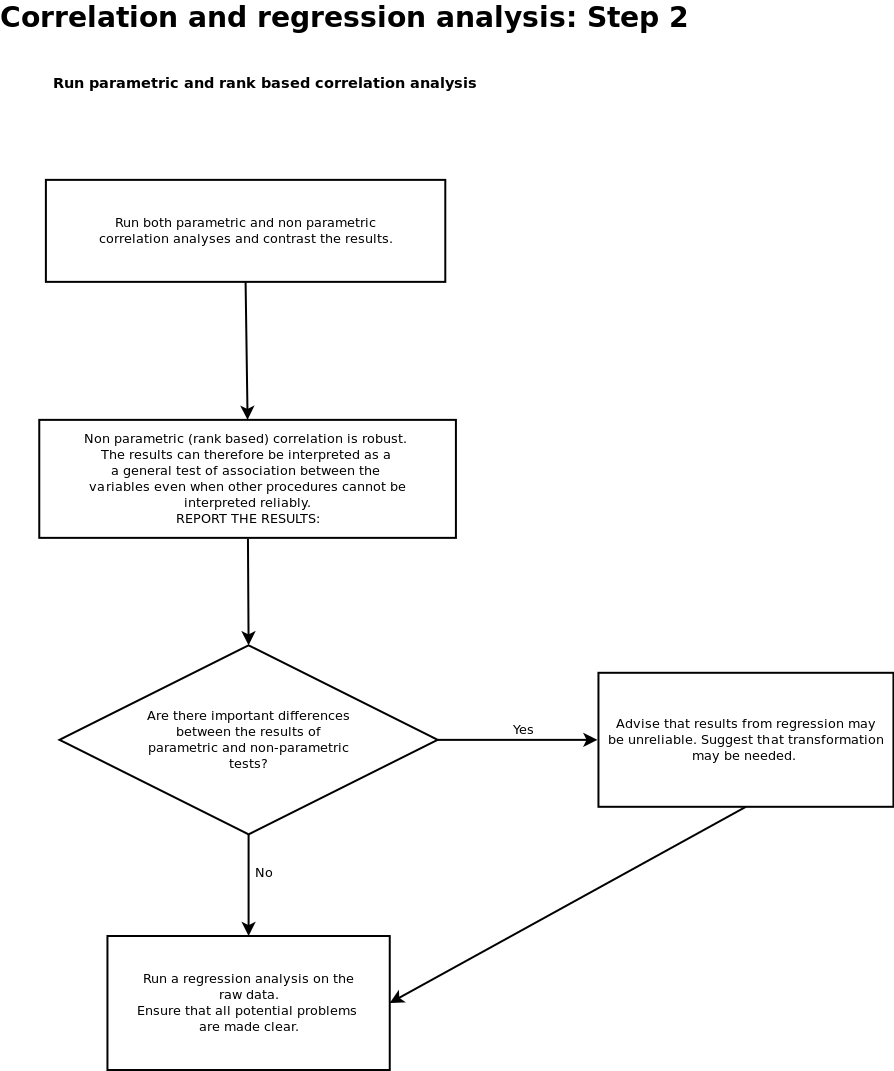## Correlation analysis

If StatsExpert (or your visual inspection) detected very serious problems due to skew or outliers then some parts of this analysis may be questionable. However non parametric rank based correlation will still be robust. So StatsExpert is now going to run both a parametric and a non parametric test for you.

StatsExpert will then try its best to flag up any issues for you after comparing the results of these tests. Read the text that it produces very carefully for advice on which test you should report.

Correlation analysis always starts with a scatterplot. You should look at this plot very carefully yourself and try to understand the relationship between the two variables by eye. Things that you should look for are

1. Evidence that the points fall along a strait line. This will result in a clear correlation.
2. The direction of the slope. If the slope rises from the origin you have a positive correlation. If the slope falls then the correlation will be negative.
3. Effects of skew and outliers. If the scatterplot is “fan shaped” this is evidence that at least one of the variables is skewed. You may need to use data transformation. StatsExpert.R will advise on this later on in the analysis.
4. Evidence of a curvilear relationship. Correlation and classical regression assume strait line relationships between your variables. If the pattern of the scatter forms a curve these methods may fail to detect a relationship even when it is very clearly there. StatsExpert.R will check this later on and advise.
``````plot(y ~ x, pch = 21, bg = 2, xlab = xtext, ylab = ytext)
``````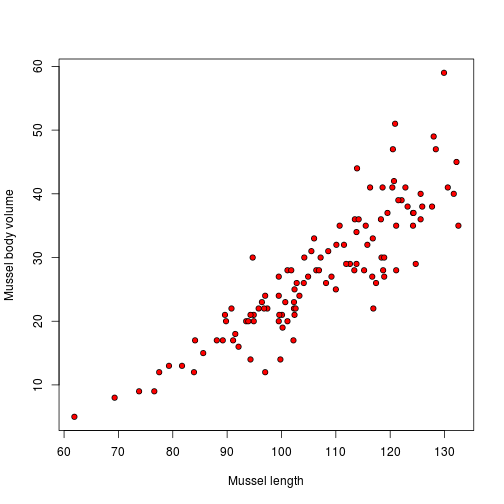There is evidence that the pattern is not ideal. This could be due to outliers or skew on the y axis

## Spearman's rank correlation

If StatsExpert has already warned you about some problems with skew or outliers you can still trust this test. The procedure is very robust as it is based on ranks. It will answer the fundamental question. Is there a relationship of some form between the two variables? Does an increase in x lead to an increase in y?
We want to know if there is a significant correlation between the ranks of the two variables. We also want some measure of the strength of this correlation. This test provides this important information even if there are problems with skew. If you have included many mistaken measurements then I am afraid that all bets are off. StatsExpert cannot know that the numbers are completely wrong. But this procedure will ignore the influence of a few erroneous outliers.

``````cor.test(x, y, method = "spearman")
``````
``````##
##  Spearman's rank correlation rho
##
## data:  x and y
## S = 26143, p-value < 2.2e-16
## alternative hypothesis: true rho is not equal to 0
## sample estimates:
##    rho
## 0.8913
##
``````

OK, so how do we interpret this output?

The p value is <0.001.
This is an extremely significant result.
We can clearly reject the null hypothesis.
The test has told us that, if all the assumptions have been met, there would be a less than one in a thousand chance of obtaining these data if the null were true. So the results are very unlikely to be attributable to chance.
As you used a non parametric test this result is quite robust.

The coefficient of determination is 0.794
This is a strong positive correlation.

The logic behind this test is shown by the scatterplot below. The test uses ranks. If this plot looks very different to the plot of the raw data then the test results will also be different.

There are no units attached to ranks, so the results are less useful in some ways. All we can say is that an increase in x leads to an increase in y.

``````xlb <- paste("Ranks of (", xtext, ")")
ylb <- paste("Ranks of (", ytext, ")")
plot(rank(y) ~ rank(x), pch = 21, bg = 2, xlab = xlb, ylab = ylb)
title(main = "Input to Spearman's test")
``````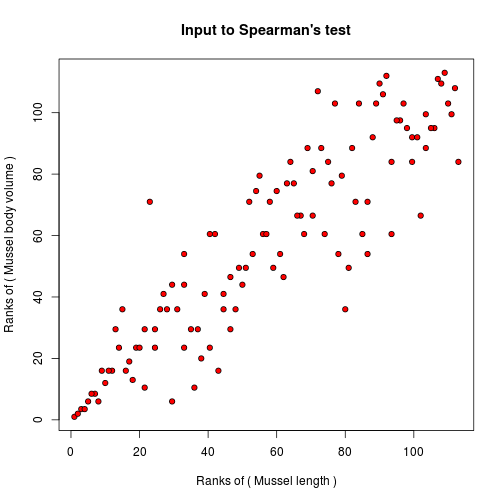## Pearson's parametric correlation

This procedure is less robust. If StatsExpert has warned you already that there are many outliers or serious skew in either of the variables then you probably should not fully trust this test. You may also have seen a difference between the scatterplots.

However if the test is more or less valid we can obtain a more detailed interpretation of the relationship using regression. So, it is very important to run both of these correlation tests and compare the results.

StatsExpert will provide some automatated text output that will help you understand the result.

``````cor.test(x, y, method = "pearson")
``````
``````##
##  Pearson's product-moment correlation
##
## data:  x and y
## t = 19.3, df = 111, p-value < 2.2e-16
## alternative hypothesis: true correlation is not equal to 0
## 95 percent confidence interval:
##  0.8271 0.9142
## sample estimates:
##    cor
## 0.8777
##
``````

OK, so how do we interpret this?

The p value is <0.001
This is a highly significant result. We can reject the null hypothesis.
The test has told us that, if all the assumptions have been met, there would be a less than one in a hundred chance of obtaining these data if the null were true. So the results are unlikely to be attributable to chance.
Because you have used a parametric test you must look carefully at the data and justify your choice of test.
You should look at the influence of any outliers and the effects of skewed data.

The coefficient of determination is 0.77
This is a strong positive correlation.

## Comparing the two tests

If the results of Spearman's test were very different to those of Pearson's then there is a strong indication that there are problems applting regression on the raw data.

If this is the case StatsExpert will first run a regression for you anyway but then will try to help to improve the results by transforming the variables for you and running a second regression analysis using the transformed variables. Let's see what StatExpert thinks is the case.

The two tests have produced very similar results.
Although there may have been some minor violations of the assumptions used in the parametric test they were not
severe enough to cause any problems. You may now use regression analysis.

## Regression analysis

The purpose of regression analysis is to quantify the relationship between your variables.
We are trying to fit a “statistical model” to the data of the form

y=a+bx

The intercept is a and b is the slope of the line. Although a regression line can be found for any data, the analalysis only really makes sense if there is a significant correlation between the variables. We have found a p-value of `<0.001` using the parametric test for correlation. We will go ahead and run the analysis. If the p-value is higher than 0.05 we will not gain anything from doing so.

So, we will plot the data again and add a line of best fit.

``````plot(y ~ x, pch = 21, bg = 2, xlab = xtext, ylab = ytext)
mod <- lm(y ~ x)
abline(mod)
``````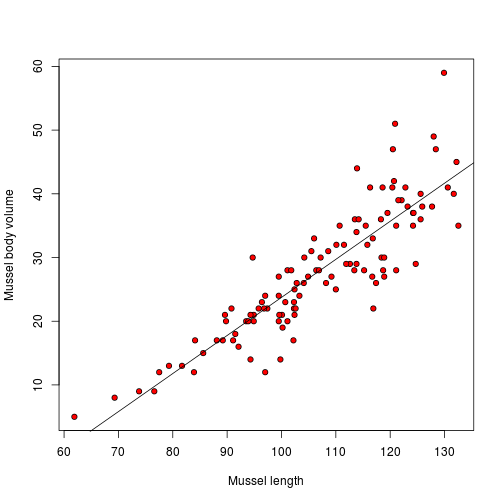``````summary(mod)
``````
``````##
## Call:
## lm(formula = y ~ x)
##
## Residuals:
##     Min      1Q  Median      3Q     Max
## -11.828  -2.672   0.147   2.235  17.404
##
## Coefficients:
##             Estimate Std. Error t value Pr(>|t|)
## (Intercept)  -36.024      3.339   -10.8   <2e-16 ***
## x              0.598      0.031    19.3   <2e-16 ***
## ---
## Signif. codes:  0 '***' 0.001 '**' 0.01 '*' 0.05 '.' 0.1 ' ' 1
##
## Residual standard error: 4.86 on 111 degrees of freedom
## Multiple R-squared: 0.77,    Adjusted R-squared: 0.768
## F-statistic:  372 on 1 and 111 DF,  p-value: <2e-16
##
``````

The most important information is that for every increase of one unit in `Mussel length` we will expect a change of `0.5975` units of `Mussel body volume`. Only you can tell if this result makes sense within the context of your study.

``````plot(y ~ x, pch = 21, bg = 2, xlab = xtext, ylab = ytext)